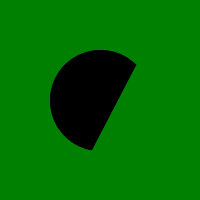Related Articles

# Wand arc() function in Python

• Last Updated : 10 May, 2020

arc() is a function present in wand.drawing module. arc() function draws an arc in the image. You’ll need to define three pairs of (x, y) coordinates. First & second pair of coordinates will be the minimum bounding rectangle, and the last pair define the starting & ending degree.

Syntax :

```wand.drawing.arc(start, end, degree)
```

Parameters :

ParameterInput TypeDescription
startsequence or (numbers.Real, numbers.Real)pair which represents starting x and y of the arc.
endsequence or (numbers.Real, numbers.Real)pair which represents ending x and y of the arc.
degreesequence or (numbers.Real, numbers.Real)pair which represents starting degree, and ending degree

Example #1:

 `# Import required objects from wand modules``from` `wand.image ``import` `Image``from` `wand.drawing ``import` `Drawing``from` `wand.color ``import` `Color`` ` `# generate object for wand.drawing``with Drawing() as draw:``    ``# set stroke color``    ``draw.stroke_color ``=` `Color(``'black'``)``    ``# set width for stroke``    ``draw.stroke_width ``=` `1``    ``# fill white color in arc``    ``draw.fill_color ``=` `Color(``'white'``)``    ``draw.arc(( ``50``, ``50``),  ``# Stating point``             ``( ``150``, ``150``),  ``# Ending point``             ``(``135``, ``-``45``))  ``# From bottom left around to top right``    ``with Image(width ``=` `100``,``               ``height ``=` `100``,``               ``background ``=` `Color(``'green'``)) as img:``        ``# draw shape on image using draw() function``        ``draw.draw(img)``        ``img.save(filename ``=``'arc.png'``)`

Output:Example #2:

Source Image:`# Import required objects from wand modules``from` `wand.image ``import` `Image``from` `wand.drawing ``import` `Drawing``from` `wand.color ``import` `Color`` ` `# generate object for wand.drawing``with Drawing() as draw:'``    ``# set stroke color``    ``draw.stroke_color ``=` `Color(``'black'``)``    ``# set width for stroke``    ``draw.stroke_width ``=` `1``    ``# fill white color in arc``    ``draw.fill_color ``=` `Color(``'white'``)``    ``draw.arc(( ``50``, ``50``),  ``# Stating point``             ``( ``150``, ``150``),  ``# Ending point``             ``(``135``, ``-``45``))  ``# From bottom left around to top right``    ``with Image(filename ``=``"gog.png"``) as img:``        ``# draw shape on image using draw() function``        ``draw.draw(img)``        ``img.save(filename ``=``'arc.png'``)`

Output:Attention geek! Strengthen your foundations with the Python Programming Foundation Course and learn the basics.

To begin with, your interview preparations Enhance your Data Structures concepts with the Python DS Course. And to begin with your Machine Learning Journey, join the Machine Learning – Basic Level Course

My Personal Notes arrow_drop_up